# Sparse least squares polynomial metamodel¶

The response of the model under consideration may be globally approximated by a multivariate polynomial. In this setup, the response is characterized by a finite number of coefficients which have to be estimated. This may be achieved by least squares. However, this method cannot be applied if the number of unknown coefficients is of similar size to the number of model evaluations, or even possibly greater. Indeed, the resulting underdetermined least squares problem would be ill-posed.
To overcome this difficulty, sparse least squares schemes may be employed (they are also known as variable selection techniques in statistics). These methods are aimed at identifying a small set of significant coefficients in the model response approximation. Eventually, a sparse polynomial response surface, i.e. a polynomial which only contains a low number of non-zero coefficients, is obtained by means of a reduced number of possibly costly model evaluations. In the sequel, we focus on sparse regression schemes based on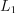-penalization.
Actually the proposed approaches do not provide only one approximation, but a collection of less and less sparse approximations. Eventually an optimal approximation may be retained with regard to a given criterion.

Context

Consider the mathematical model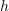of a physical system depending on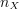input parameters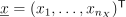. Note that these input variables are assumed to be deterministic in this section. The model response may be approximated by a polynomial as follows:

(1)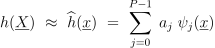Let us consider a set of values taken by the input vector (i.e. an experimental design)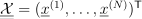as well as the vector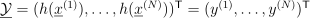of the corresponding model evaluations. It is assumed that the number of terms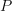in the polynomial basis is of similar size to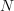, or even possibly significantly larger than. In such a situation it is not possible to compute the polynomial coefficients by ordinary least squares, since the corresponding system is ill-posed. Methods that may be employed as an alternative are outlined in the sequel.
LASSO
The so-called LASSO (for Least absolute shrinkage and selection operator) method is based on a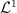-penalized regression as follows:

(2)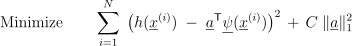where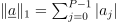and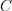is a non negative constant. A nice feature of LASSO is that it provides a sparse metamodel, i.e. it discards insignificant variables from the set of predictors. The obtained response surface is all the sparser since the value of the tuning parameteris high.

For a given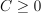, the solving procedure may be implemented via quadratic programming. Obtaining the whole set of coefficient estimates forvarying from 0 to a maximum value may be computationally expensive though since it requires solving the optimization problem for a fine grid of values of.
Forward stagewise regression

Another procedure, known as forward stagewise regression, appears to be different from LASSO, but turns out to provide similar results. The procedure first picks the predictor that is most correlated with the vector of observations. However, the value of the corresponding coefficient is only increased by a small amount. Then the predictor with largest correlation with the current residual (possible the same term as in the previous step) is picked, and so on. Let us introduce the vector notation: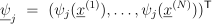The forward stagewise algorithm is outlined below:

1. Start with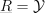and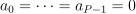.

2. Find the predictor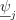that is most correlated with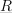.

3. Update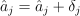, where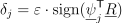.

4. Update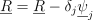and repeats Steps 2 and 3 until no predictor has any correlation with.

Note that parameterhas to be set equal to a small value in practice, say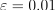. This procedure is known to be more stable than traditional stepwise regression.
Least Angle Regression

Least Angle Regression (LAR) may be viewed as a version of forward stagewise that uses mathematical derivations to speed up the computations. Indeed, instead of taking many small steps with the basis term most correlated with current residual, the related coefficient is directly increased up to the point where some other basis predictor has as much correlation with. Then the new predictor is entered, and the procedure is continued. The LARS algorithm is detailed below:

1. Initialize the coefficients to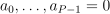. Set the initial residual equal to the vector of observations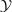.

2. Find the vectorwhich is most correlated with the current residual.

3. Move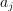from 0 toward the least-square coefficient of the current residual on, until some other predictor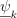has as much correlation with the current residual as does.

4. Move jointly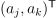in the direction defined by their joint least-square coefficient of the current residual on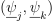, until some other predictor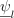has as much correlation with the current residual.

5. Continue this way until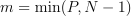predictors have been entered.

Steps 2 and 3 correspond to a “move” of the active coefficients toward their least-square value. It corresponds to an updating of the form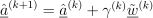. Vector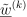and coefficient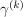are referred to as the LARS descent direction and step, respectively. Both quantities may be derived algebraically.
Note that if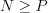, then the last step of LARS provides the ordinary least-square solution. It is shown that LARS is noticeably efficient since it only requires the computational cost of ordinary least-square regression onpredictors for producing a collection ofmetamodels.
LASSO and Forward Stagewise as variants of LARS

It has been shown that with only one modification, the LARS procedure provides in one shot the entire paths of LASSO solution coefficients as the tuning parameterin (2) is increased from 0 up to a maximum value. The modified algorithm is as follows:

• Run the LARS procedure from Steps 1 to 4.

• If a non zero coefficient hits zero, discard it from the current metamodel and recompute the current joint least-square direction.

• Continue this way untilpredictors have been entered.

Note that the LAR-based LASSO procedure may take more thansteps since the predictors are allowed to be discarded and introduced later again into the metamodel. In a similar fashion, a limiting version of the forward stagewise method when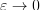may be obtained by slightly modifying the original LARS algorithm. In the literature, one commonly uses the label LARS when referring to all these LAR-based algorithms (with S referring to Stagewise and LASSO).
Selection of the optimal LARS solution

The LAR-based approaches (i.e. original LAR, LASSO and forward stagewise) provide a collection of less and less sparse PC approximations. The accuracy of each PC metamodel may be assessed by cross validation. Eventually the PC representation associated with the lowest error estimate is retained.